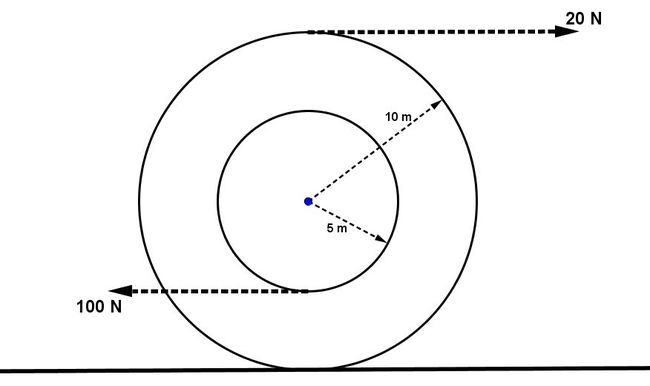# Acceleration of a cotton reelTwo forces of $100N$ and $20N$ act on a cotton reel of inner radius $5 \text{ m}$ and outer radius $10 \text{ m}$ as shown in the figure above. The reel is performing pure rolling motion. The surface produces sufficient necessary friction for pure rolling of the reel. Find the linear acceleration of the reel (in $\text{ m/s}^2$).

Details and Assumptions

• The mass of the cotton reel is $10 \text{ kg}$.

• The moment of inertia of the reel about central axis is $1000 \text{ kg m}^2$.

×